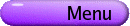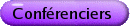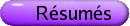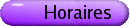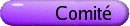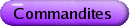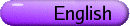Conférenciers pléniers[PDF] BRENT DAVIS, University of British Columbia, 2125 Main Mall, Vancouver, BC, V6T 1Z4 Mathematics-for-Teaching: Where are we with all of this? [PDF] Over the past decade, mathematics-for-teaching (MfT) has emerged as the most prominent and popular topic in mathematics education research. In this presentation, I offer a partial review of research orientations and findings that give shape to current discussions and investigations. In the process, I flag what I think to be some of the more promising avenues. I also attempt to highlight a few of the pragmatic implications for Departments of Mathematics and Faculties of Education. DMITRY DOLGOPYAT, Pennsylvania State University, University Park, PA, 16802, USA Fermi acceleration [PDF] Fermi acceleration is a mechanism, first suggested by Enrico Fermi in 1949, to explain heating of particles in cosmic rays. Fermi studied charged particles being reflected by the moving interstellar magnetic field and either gaining or losing energy, depending on whether the "magnetic mirror" is approaching or receding. In a typical environment, Fermi argued, the probability of a head-on collision is greater than a head-tail collision, so particles would, on average, be accelerated. Since then Fermi acceleration has been used to explain a number of natural phenomena and several simple mathematical models demonstrating Fermi acceleration have been proposed. We describe these models and explain why they do or do not exhibit Fermi acceleration. We also mention some models where the answer is not known. DIMITRI SHLYAKHTENKO, University of California at Los Angeles L2 Invariants, Free Probability and Operator Algebras [PDF] The Cheeger-Gromov L2 Betti numbers of a discrete group are numerical invariants, going back to Atiyah's work on the equivariant Atiyah-Singer index theorem. On the other hand, Voiculescu has introduced another discrete group invariant, coming from his free probability theory, called the free entropy dimension. Very roughly, this number measures the "asymptotic dimensions" of the sets of approximate embeddings of a group into unitary matrices. We describe our joint work with A. Connes and I. Mineyev that has provided a connection between these numbers. Both of these approaches (operator algebra versions of L2 Betti numbers and Voiculescu's free entropy dimension) are attempts to arrive at the von Neumann algebra analog of the theory of L2 Betti numbers (Gaboriau) and of free entropy dimension for measurable equivalence relations. KAREN SMITH, University of Michigan, Ann Arbor, MI Uniform Results in Algebra and Geometry via Multiplier Ideals [PDF] In recent years, there has been a flurry of questions about uniform behavior in commutative algebra and algebraic geometry. Multiplier ideals have had a tremendous impact in solving many of these questions. Multiplier ideals can be defined in three rather different ways. Originally defined by analysts, they are functions in some L2-space. For algebraic geometers, they are defined via resolutions of singularities. For commutative algebraists, they are defined in rings of prime characteristic using tight closure. In this talk, we will discuss a few of the diverse problems about uniform behavior in algebra and geometry that have been solved using multiplier ideals, as well as the different perspectives from which multiplier ideals can be viewed. SUSAN TOLMAN, University of Illinois at Urbana-Champaign Group actions and the symplectomorphism group [PDF] Much progress has been made in recent years studying the group of diffeomorphisms which preserve a symplectic form. We will discuss recent advances in this question, focussing on the relationship between this group and its compact subgroups. In particular, we will consider how to use these subgroups to gain information about the group itself, and ways in which it behaves (or does not behave) like a compact group. SHMUEL WEINBERGER, University of Chicago Quantitative Aspects of Topology [PDF] In the popular imagination, there is a strict dichotomy between the quantitative and the qualitative and Topology is the epitome of the qualitative. However, many problems, both theoretical and applied, have suggested a need for topology to encompass estimates and to grow to include discontinuous maps. I will try to explain some of these via examples taken from problems like estimating solutions to equations, the influence of the fundamental group on the geometry/topology of manifolds, and recent methods of data analysis.